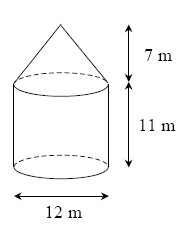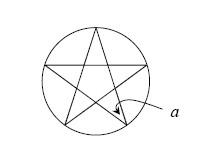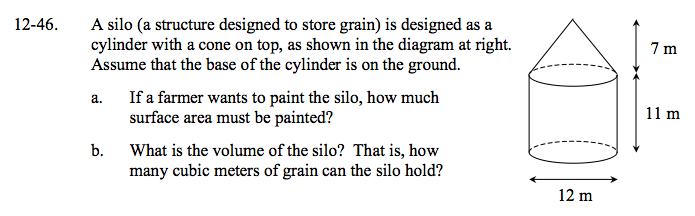### Home > GC > Chapter 12 > Lesson 12.1.5 > Problem12-46

12-46.
1. A silo (a structure designed to store grain) is designed as a cylinder with a cone on top, as shown in the diagram below. Assume that the base of the cylinder is on the ground. Homework Help ✎1. If a farmer wants to paint the silo, how much surface area must be painted?

2. What is the volume of the silo? That is, how many cubic meters of grain can the silo hold?Find the lateral area of the cone and the lateral area of the cylinder, then find their sum.

The slant height of the cone ≈ 9.22 m
Lateral Area (cone) = 6π(9.22) ≈ 173.78
Lateral Area (cylinder) = 12π(11) = 132π ≈ 414.69
173.78 + 414.69 = 588.47 m²

Find the volume of the cone and the volume of the cylinder, then find their sum.